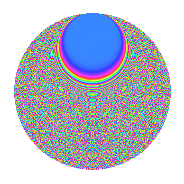# Properties

 Label 3234.2.bwLevel 3234 Weight 2 Character orbit bw Rep. character $$\chi_{3234}(89,\cdot)$$ Character field $$\Q(\zeta_{42})$$ Dimension 2256 Sturm bound 1344

# Related objects

## Defining parameters

 Level: $$N$$ $$=$$ $$3234 = 2 \cdot 3 \cdot 7^{2} \cdot 11$$ Weight: $$k$$ $$=$$ $$2$$ Character orbit: $$[\chi]$$ $$=$$ 3234.bw (of order $$42$$ and degree $$12$$) Character conductor: $$\operatorname{cond}(\chi)$$ $$=$$ $$147$$ Character field: $$\Q(\zeta_{42})$$ Sturm bound: $$1344$$

## Dimensions

The following table gives the dimensions of various subspaces of $$M_{2}(3234, [\chi])$$.

Total New Old
Modular forms 8160 2256 5904
Cusp forms 7968 2256 5712
Eisenstein series 192 0 192

## Trace form

 $$2256q - 188q^{4} - 28q^{6} - 16q^{7} - 32q^{9} + O(q^{10})$$ $$2256q - 188q^{4} - 28q^{6} - 16q^{7} - 32q^{9} + 24q^{15} + 188q^{16} + 24q^{19} - 16q^{21} + 12q^{24} + 196q^{25} - 8q^{28} - 8q^{30} - 24q^{31} + 20q^{36} + 68q^{37} + 12q^{39} + 48q^{42} - 16q^{43} - 48q^{45} + 312q^{46} + 40q^{49} + 12q^{51} - 32q^{52} + 36q^{54} + 60q^{57} + 52q^{58} + 12q^{60} - 112q^{61} + 56q^{63} + 376q^{64} + 8q^{67} - 32q^{70} - 48q^{73} - 132q^{75} + 32q^{81} - 24q^{82} - 80q^{84} - 128q^{85} + 36q^{87} - 84q^{90} + 16q^{91} + 536q^{93} + 48q^{94} + 12q^{96} + O(q^{100})$$

## Decomposition of $$S_{2}^{\mathrm{new}}(3234, [\chi])$$ into newform subspaces

The newforms in this space have not yet been added to the LMFDB.

## Decomposition of $$S_{2}^{\mathrm{old}}(3234, [\chi])$$ into lower level spaces

$$S_{2}^{\mathrm{old}}(3234, [\chi]) \cong$$ $$S_{2}^{\mathrm{new}}(147, [\chi])$$$$^{\oplus 4}$$$$\oplus$$$$S_{2}^{\mathrm{new}}(294, [\chi])$$$$^{\oplus 2}$$$$\oplus$$$$S_{2}^{\mathrm{new}}(1617, [\chi])$$$$^{\oplus 2}$$

## Hecke characteristic polynomials

There are no characteristic polynomials of Hecke operators in the database